# CGNM: Cluster Gauss-Newton Method

library(CGNM)
library(knitr)

# When and when not to use CGNM

## Use CGNM

• Wish to fit relatively complex parameterized model/curve/function to the data; however, unsure of the appropriate initial guess (e.g., “start” argument in nls function). => CGNM searches the parameter from multiple initial guess so that the use can specify rough initial range instead of a point initial guess.
• When the practical identifiability (if there is only one best fit parameter or not) is unknown. => CGNM will find multiple set of best fit parameters; hence if the parameter is not practically identifiable then the multiple best-fit parameters found by CGNM will not converge to a point.
• When the model is a blackbox (i.e., cannot be explicitly/easily write out as a “formula”) and the model may not be continuous with respect to the parameter. => CGNM makes minimum assumptions on the model so all user need to provide is a function that takes the model parameter and simulation (and what happen in the function, CGNM does not care).

## Not to use CGNM

• When the you already know where approximately the best fit parameter is and you just need to find one best fit parameter. => Simply use nls and that will be faster.
• When the model is relatively simple and computation cost is not an issue. => CGNM is made to save the number of model evaluation during the parameter estimation, so may not see much advantage compared to the conventional multi-start methods (e.g.repeatedly using nls from various “start”).

# How to use CGNM

To illustrate the use of CGNM here we illustrate how CGNM can be used to estimate two sets of the best fit parameters of the pharmacokinetics model when the drug is administered orally (known as flip-flop kinetics).

## Prepare the model ($$\boldsymbol f$$)

model_function=function(x){

observation_time=c(0.1,0.2,0.4,0.6,1,2,3,6,12)
Dose=1000
F=1

ka=x
V1=x
CL_2=x
t=observation_time

Cp=ka*F*Dose/(V1*(ka-CL_2/V1))*(exp(-CL_2/V1*t)-exp(-ka*t))

log10(Cp)
}

## Prepare the data ($$\boldsymbol y^*$$)

observation=log10(c(4.91, 8.65, 12.4, 18.7, 24.3, 24.5, 18.4, 4.66, 0.238))

## Run Cluster_Gauss_Newton_method

Here we have specified the upper and lower range of the initial guess.

CGNM_result=Cluster_Gauss_Newton_method(nonlinearFunction=model_function,
targetVector = observation,
initial_lowerRange =rep(0.01,3),initial_upperRange =  rep(100,3),lowerBound = rep(0,3), saveLog=TRUE, num_minimizersToFind = 500, ParameterNames = c("Ka","V1","CL"))
#>  "checking if the nonlinearFunction can be evaluated at the initial_lowerRange"
#>  "Evaluation Successful"
#>  "checking if the nonlinearFunction can be evaluated at the initial_upperRange"
#>  "Evaluation Successful"
#>  "checking if the nonlinearFunction can be evaluated at the (initial_upperRange+initial_lowerRange)/2"
#>  "Evaluation Successful"
#>  "CGNM iteration should finish before: 2023-05-19 19:23:51.722006"
#>  "Generating initial cluster. 493 out of 500 done"
#>  "Generating initial cluster. 500 out of 500 done"
#>  "Iteration:1  Median sum of squares residual=5.84753446043647"
#>  "Rough estimation of remaining computation time: 0.6 min"
#>  "CGNM iteration estimated to finish at: 2023-05-19 19:24:11.360814"
#>  "Iteration:2  Median sum of squares residual=2.29986581593537"
#>  "Iteration:3  Median sum of squares residual=1.0178329859486"
#>  "Iteration:4  Median sum of squares residual=0.923920239882494"
#>  "Iteration:5  Median sum of squares residual=0.648310653160494"
#>  "Iteration:6  Median sum of squares residual=0.0833997901388448"
#>  "Iteration:7  Median sum of squares residual=0.00804223585482565"
#>  "Iteration:8  Median sum of squares residual=0.00735541853841457"
#>  "Iteration:9  Median sum of squares residual=0.00734951504875528"
#>  "Iteration:10  Median sum of squares residual=0.00734923479686116"
#>  "Iteration:11  Median sum of squares residual=0.00734923414772126"
#>  "CGNM iteration estimated to finish at: 2023-05-19 19:24:10.751123"
#>  "Iteration:12  Median sum of squares residual=0.00734923408294392"
#>  "Iteration:13  Median sum of squares residual=0.0073492340825258"
#>  "Iteration:14  Median sum of squares residual=0.00734923408245357"
#>  "Iteration:15  Median sum of squares residual=0.00734923408238969"
#>  "Iteration:16  Median sum of squares residual=0.0073492340823856"
#>  "Iteration:17  Median sum of squares residual=0.0073492340823823"
#>  "Iteration:18  Median sum of squares residual=0.00734923408238179"
#>  "Iteration:19  Median sum of squares residual=0.00734923408238119"
#>  "Iteration:20  Median sum of squares residual=0.00734923408238094"
#>  "Iteration:21  Median sum of squares residual=0.00734923408238088"
#>  "CGNM iteration estimated to finish at: 2023-05-19 19:24:10.915151"
#>  "Iteration:22  Median sum of squares residual=0.00734923408238085"
#>  "Iteration:23  Median sum of squares residual=0.00734923408238084"
#>  "Iteration:24  Median sum of squares residual=0.00734923408238084"
#>  "Iteration:25  Median sum of squares residual=0.00734923408238083"
#>  "CGNM computation time:  0.6 min"

## Obtain the approximate minimizers

kable(head(acceptedApproximateMinimizers(CGNM_result)))
Ka V1 CL
0.5178956 10.66084 9.877326
0.5178956 10.66084 9.877326
0.5178956 10.66084 9.877326
0.5178953 10.66083 9.877326
0.5178956 10.66084 9.877326
0.9265056 19.07204 9.877326
kable(table_parameterSummary(CGNM_result))
CGNM: Minimum 25 percentile Median 75 percentile Maximum
Ka 0.5178833 0.5178956 0.5178956 0.9265056 0.9265267
V1 10.6603944 10.6608371 10.6608375 19.0720415 19.0722210
CL 9.8771738 9.8773257 9.8773257 9.8773258 9.8777329

## Can run residual resampling bootstrap analyses using CGNM as well

CGNM_bootstrap=Cluster_Gauss_Newton_Bootstrap_method(CGNM_result, nonlinearFunction=model_function)
#>  "checking if the nonlinearFunction can be evaluated at the initial_lowerRange"
#>  "Evaluation Successful"
#>  "checking if the nonlinearFunction can be evaluated at the initial_upperRange"
#>  "Evaluation Successful"
#>  "checking if the nonlinearFunction can be evaluated at the (initial_upperRange+initial_lowerRange)/2"
#>  "Evaluation Successful"
#>  "CGNM iteration should finish before: 2023-05-19 19:24:11.104984"
#>  "Generating initial cluster. 200 out of 200 done"
#>  "Iteration:1  Median sum of squares residual=0.0113846607433386"
#>  "Rough estimation of remaining computation time: 0.1 min"
#>  "CGNM iteration estimated to finish at: 2023-05-19 19:24:14.971681"
#>  "Iteration:2  Median sum of squares residual=0.0108323904824184"
#>  "Iteration:3  Median sum of squares residual=0.0107301370495941"
#>  "Iteration:4  Median sum of squares residual=0.0107301031286924"
#>  "Iteration:5  Median sum of squares residual=0.010730096842903"
#>  "Iteration:6  Median sum of squares residual=0.010730096802742"
#>  "Iteration:7  Median sum of squares residual=0.0107300947194723"
#>  "Iteration:8  Median sum of squares residual=0.0107300947194723"
#>  "Iteration:9  Median sum of squares residual=0.0107300947194723"
#>  "Iteration:10  Median sum of squares residual=0.0107300947194723"
#>  "Iteration:11  Median sum of squares residual=0.0107300947194723"
#>  "CGNM iteration estimated to finish at: 2023-05-19 19:24:14.911324"
#>  "Iteration:12  Median sum of squares residual=0.0107300947186618"
#>  "Iteration:13  Median sum of squares residual=0.0107300947186618"
#>  "Iteration:14  Median sum of squares residual=0.0107300947186618"
#>  "Iteration:15  Median sum of squares residual=0.0107300945603149"
#>  "Iteration:16  Median sum of squares residual=0.0107300945603149"
#>  "Iteration:17  Median sum of squares residual=0.0107300945603141"
#>  "Iteration:18  Median sum of squares residual=0.0107300945211936"
#>  "Iteration:19  Median sum of squares residual=0.0107300945211936"
#>  "Iteration:20  Median sum of squares residual=0.0107300945211936"
#>  "Iteration:21  Median sum of squares residual=0.0107300945211936"
#>  "CGNM iteration estimated to finish at: 2023-05-19 19:24:14.873109"
#>  "Iteration:22  Median sum of squares residual=0.0107300945211936"
#>  "Iteration:23  Median sum of squares residual=0.0107300945211936"
#>  "Iteration:24  Median sum of squares residual=0.0107300945211936"
#>  "Iteration:25  Median sum of squares residual=0.0107300945211936"
#>  "CGNM computation time:  0.1 min"
kable(table_parameterSummary(CGNM_bootstrap))
CGNM Bootstrap: Minimum 25 percentile Median 75 percentile Maximum RSE (%)
Ka 0.4788361 0.5181835 0.5371069 0.907249 1.114479 29.971990
V1 9.1433389 10.5894825 11.2640031 18.654266 22.308660 29.661392
CL 9.4211816 9.6443352 9.8001175 9.980728 10.908495 2.812164

## Visualize the CGNM modelfit analysis result

To use the plot functions the user needs to manually load ggplot2.

library(ggplot2)

### Inspect the distribution of SSR of approximate minimizers found by CGNM

Despite the robustness of the algorithm not all approximate minimizers converge so here we visually inspect to see how many of the approximate minimizers we consider to have the similar SSR to the minimum SSR. Currently the algorithm automatically choose “acceptable” approximate minimizer based on Grubbs’ Test for Outliers. If for whatever the reason this criterion is not satisfactly the users can manually set the indicies of the acceptable approximat minimizers.

plot_Rank_SSR(CGNM_result)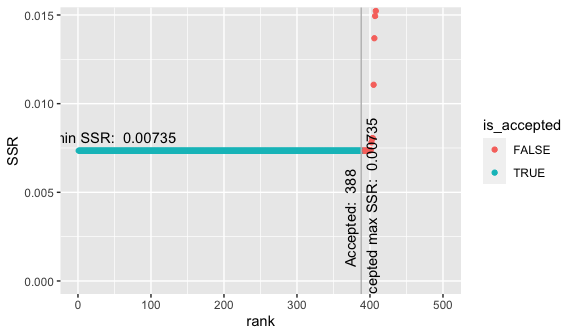plot_paraDistribution_byHistogram(CGNM_bootstrap, bins = 50)+scale_x_continuous(trans="log10")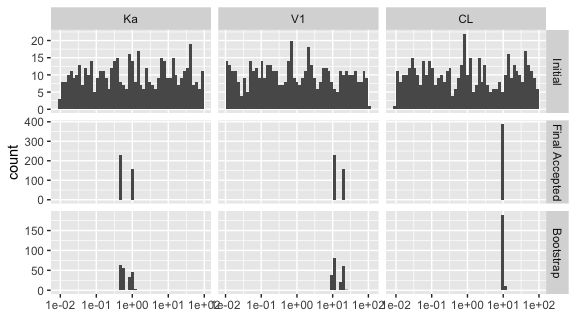### visually inspect goodness of fit of top 50 approximate minimizers

plot_goodnessOfFit(CGNM_result, plotType = 1, independentVariableVector = c(0.1,0.2,0.4,0.6,1,2,3,6,12), plotRank = seq(1,50))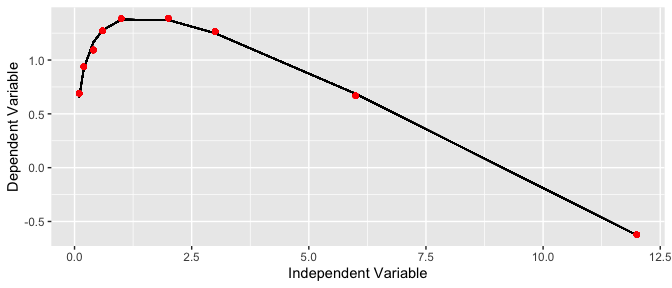### plot model prediction with uncertainties based on residual resampling bootstrap analysis

plot_goodnessOfFit(CGNM_bootstrap, plotType = 1, independentVariableVector = c(0.1,0.2,0.4,0.6,1,2,3,6,12))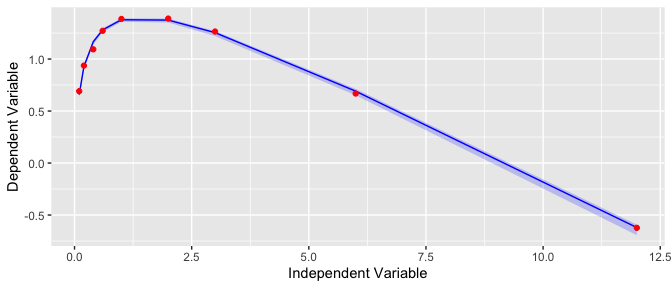### plot profile likelihood

plot_profileLikelihood(c("CGNM_log","CGNM_log_bootstrap"))+scale_x_continuous(trans="log10")
#>  "log saved in /private/var/folders/n8/mrjc8j9j45x93m7t_hmvg9nm0000gn/T/RtmpjHO9Sk/Rbuild21ab7dd69f95/CGNM/vignettes/CGNM_log is used to draw SSR/likelihood surface"
#> Warning in prepSSRsurfaceData(logLocation, ParameterNames,
#> /private/var/folders/n8/mrjc8j9j45x93m7t_hmvg9nm0000gn/T/RtmpjHO9Sk/Rbuild21ab7dd69f95/CGNM/vignettes/CGNM_log
#> is not the same as
#> /private/var/folders/n8/mrjc8j9j45x93m7t_hmvg9nm0000gn/T/RtmpjHO9Sk/Rbuild21ab7dd69f95/CGNM/vignettes/CGNM_log
#>  "log saved in /private/var/folders/n8/mrjc8j9j45x93m7t_hmvg9nm0000gn/T/RtmpjHO9Sk/Rbuild21ab7dd69f95/CGNM/vignettes/CGNM_log_bootstrap is used to draw SSR/likelihood surface"
#> Warning in prepSSRsurfaceData(logLocation, ParameterNames,
#> /private/var/folders/n8/mrjc8j9j45x93m7t_hmvg9nm0000gn/T/RtmpjHO9Sk/Rbuild21ab7dd69f95/CGNM/vignettes/CGNM_log_bootstrap
#> is not the same as
#> /private/var/folders/n8/mrjc8j9j45x93m7t_hmvg9nm0000gn/T/RtmpjHO9Sk/Rbuild21ab7dd69f95/CGNM/vignettes/CGNM_log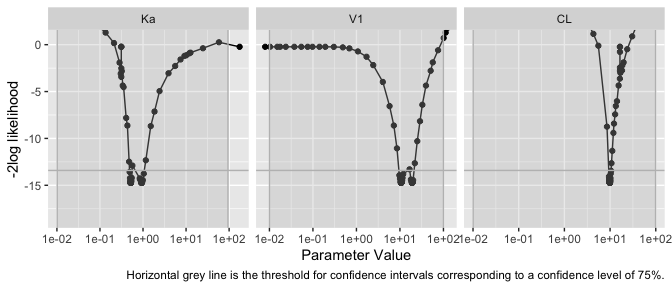kable(table_profileLikelihoodConfidenceInterval(c("CGNM_log","CGNM_log_bootstrap"), alpha = 0.25))
#>  "log saved in /private/var/folders/n8/mrjc8j9j45x93m7t_hmvg9nm0000gn/T/RtmpjHO9Sk/Rbuild21ab7dd69f95/CGNM/vignettes/CGNM_log is used to draw SSR/likelihood surface"
#> Warning in prepSSRsurfaceData(logLocation, ParameterNames,
#> /private/var/folders/n8/mrjc8j9j45x93m7t_hmvg9nm0000gn/T/RtmpjHO9Sk/Rbuild21ab7dd69f95/CGNM/vignettes/CGNM_log
#> is not the same as
#> /private/var/folders/n8/mrjc8j9j45x93m7t_hmvg9nm0000gn/T/RtmpjHO9Sk/Rbuild21ab7dd69f95/CGNM/vignettes/CGNM_log
#>  "log saved in /private/var/folders/n8/mrjc8j9j45x93m7t_hmvg9nm0000gn/T/RtmpjHO9Sk/Rbuild21ab7dd69f95/CGNM/vignettes/CGNM_log_bootstrap is used to draw SSR/likelihood surface"
#> Warning in prepSSRsurfaceData(logLocation, ParameterNames,
#> /private/var/folders/n8/mrjc8j9j45x93m7t_hmvg9nm0000gn/T/RtmpjHO9Sk/Rbuild21ab7dd69f95/CGNM/vignettes/CGNM_log_bootstrap
#> is not the same as
#> /private/var/folders/n8/mrjc8j9j45x93m7t_hmvg9nm0000gn/T/RtmpjHO9Sk/Rbuild21ab7dd69f95/CGNM/vignettes/CGNM_log
Parameter Name 25 percentile 75 percentile
Ka 0.481699 1.101842
V1 9.113612 21.018241
CL 9.014966 10.622573

### plot profile likelihood surface

plot_2DprofileLikelihood(CGNM_result, showInitialRange=FALSE, alpha = 0.05)+scale_x_continuous(trans="log10")+scale_y_continuous(trans="log10")
#>  "log saved in /private/var/folders/n8/mrjc8j9j45x93m7t_hmvg9nm0000gn/T/RtmpjHO9Sk/Rbuild21ab7dd69f95/CGNM/vignettes/CGNM_log is used to draw SSR/likelihood surface"
#> Warning in prepSSRsurfaceData(logLocation, ParameterNames,
#> /private/var/folders/n8/mrjc8j9j45x93m7t_hmvg9nm0000gn/T/RtmpjHO9Sk/Rbuild21ab7dd69f95/CGNM/vignettes/CGNM_log
#> is not the same as
#> /private/var/folders/n8/mrjc8j9j45x93m7t_hmvg9nm0000gn/T/RtmpjHO9Sk/Rbuild21ab7dd69f95/CGNM/vignettes/CGNM_log
#>  "log saved in /private/var/folders/n8/mrjc8j9j45x93m7t_hmvg9nm0000gn/T/RtmpjHO9Sk/Rbuild21ab7dd69f95/CGNM/vignettes/CGNM_log_bootstrap is used to draw SSR/likelihood surface"
#> Warning in prepSSRsurfaceData(logLocation, ParameterNames,
#> /private/var/folders/n8/mrjc8j9j45x93m7t_hmvg9nm0000gn/T/RtmpjHO9Sk/Rbuild21ab7dd69f95/CGNM/vignettes/CGNM_log_bootstrap
#> is not the same as
#> /private/var/folders/n8/mrjc8j9j45x93m7t_hmvg9nm0000gn/T/RtmpjHO9Sk/Rbuild21ab7dd69f95/CGNM/vignettes/CGNM_log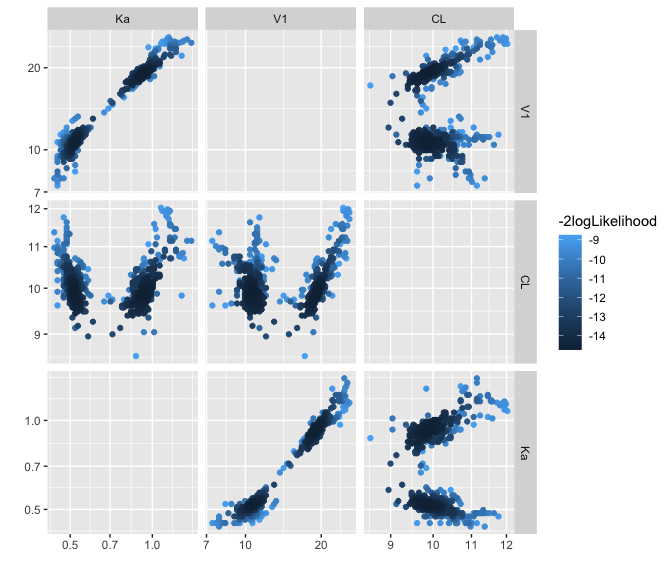## Parallel computation

Cluster Gauss Newton method implementation in CGNM package (above version 0.6) can use nonlinear function that takes multiple input vectors stored in matrix (each column as the input vector) and output matrix (each column as the output vector). This implementation was to be used to parallelize the computation. See below for the examples of parallelized implementation in various hardware. Cluster Gauss Newton method is embarrassingly parallelizable so the computation speed is almost proportional to the number of computation cores used especially for the nonlinear functions that takes time to compute (e.g. models with numerical method to solve a large system of ODEs).

model_matrix_function=function(X){
Y_list=lapply(split(X, rep(seq(1:nrow(X)),ncol(X))), model_function)
Y=t(matrix(unlist(Y_list),ncol=length(Y_list)))
}

testX=t(matrix(c(rep(0.01,3),rep(10,3),rep(100,3)), nrow = 3))
print("testX")
#>  "testX"
print(testX)
#>       [,1]  [,2]  [,3]
#> [1,] 1e-02 1e-02 1e-02
#> [2,] 1e+01 1e+01 1e+01
#> [3,] 1e+02 1e+02 1e+02
print("model_matrix_function(testX)")
#>  "model_matrix_function(testX)"
print(model_matrix_function(testX))
#>           [,1]      [,2]     [,3]      [,4]      [,5]      [,6]       [,7]
#> [1,] 1.9782455 2.2578754 2.517166 2.6529261 2.7982741 2.9311513  2.9684634
#> [2,] 1.7756978 1.8804296 1.860008 1.7832148 1.6114094 1.1771685  0.7428740
#> [3,] 0.9609136 0.9175059 0.830647 0.7437881 0.5700703 0.1357758 -0.2985186
#>            [,8]      [,9]
#> [1,]  2.9771626  2.952246
#> [2,] -0.5600094 -3.165776
#> [3,] -1.6014021 -4.207169

print("model_matrix_function(testX)-rbind(model_function(testX[1,]),model_function(testX[2,]),model_function(testX[3,]))")
#>  "model_matrix_function(testX)-rbind(model_function(testX[1,]),model_function(testX[2,]),model_function(testX[3,]))"
print(model_matrix_function(testX)-rbind(model_function(testX[1,]),model_function(testX[2,]),model_function(testX[3,])))
#>      [,1] [,2] [,3] [,4] [,5] [,6] [,7] [,8] [,9]
#> [1,]    0    0    0    0    0    0    0    0    0
#> [2,]    0    0    0    0    0    0    0    0    0
#> [3,]    0    0    0    0    0    0    0    0    0

### an example of parallel implementation for Mac using parallel package


# library(parallel)
#
# obsLength=length(observation)
#
## Given CGNM searches through wide range of parameter combination, it can encounter
## parameter combinations that is not feasible to evaluate. This try catch function
## is implemented within CGNM for regular functions but for the matrix functions
## user needs to implement outside of CGNM
#
# modelFunction_tryCatch=function(x_in){
#  out=tryCatch({model_function(x_in)},
#               error=function(cond) {rep(NA, obsLength)}
#  )
#  return(out)
# }
#
#  model_matrix_function=function(X){
#   Y_list=mclapply(split(X, rep(seq(1:nrow(X)),ncol(X))), modelFunction_tryCatch,mc.cores = (parallel::detectCores()-1), mc.preschedule = FALSE)
#
#   Y=t(matrix(unlist(Y_list),ncol=length(Y_list)))
#
#   return(Y)
#  }

### an example of parallel implementation for Windows using foreach and doParllel packages

#library(foreach)
#library(doParallel)

#numCore=8
#registerDoParallel(numCore-1)
#cluster=makeCluster(numCore-1, type = "PSOCK")
#registerDoParallel(cl=cluster)

# obsLength=length(observation)

## Given CGNM searches through wide range of parameter combination, it can encounter
## parameter combinations that is not feasible to evaluate. This try catch function
## is implemented within CGNM for regular functions but for the matrix functions
## user needs to implement outside of CGNM

# modelFunction_tryCatch=function(x_in){
#  out=tryCatch({model_function(x_in)},
#               error=function(cond) {rep(NA, obsLength)}
#  )
#  return(out)
# }

#model_matrix_function=function(X){
#  Y_list=foreach(i=1:dim(X), .export = c("model_function", "modelFunction_tryCatch"))%dopar%{ #make sure to include all related functions in .export and all used packages in .packages for more information read documentation of dopar
#      modelFunction_tryCatch((X[i,]))
#    }

#  Y=t(matrix(unlist(Y_list),ncol=length(Y_list)))
#}
CGNM_result=Cluster_Gauss_Newton_method(nonlinearFunction=model_matrix_function,
targetVector = observation,
initial_lowerRange =rep(0.01,3),initial_upperRange =  rep(100,3),lowerBound = rep(0,3), saveLog=TRUE, num_minimizersToFind = 500, ParameterNames = c("Ka","V1","CL"))
#>  "nonlinearFunction is given as matrix to matrix function"
#>  "NonlinearFunction evaluation at initial_lowerRange Successful."
#>  "NonlinearFunction evaluation at (initial_upperRange+initial_lowerRange)/2 Successful."
#>  "NonlinearFunction evaluation at initial_upperRange Successful."
#>  "CGNM iteration should finish before: 2023-05-19 19:24:18.020459"
#> Warning in dir.create(saveFolderName): 'CGNM_log' already exists
#>  "Generating initial cluster. 496 out of 500 done"
#>  "Generating initial cluster. 500 out of 500 done"
#>  "Iteration:1  Median sum of squares residual=5.91808789144101"
#>  "Rough estimation of remaining computation time: 0.6 min"
#>  "CGNM iteration estimated to finish at: 2023-05-19 19:24:56.541781"
#>  "Iteration:2  Median sum of squares residual=2.2485680196151"
#>  "Iteration:3  Median sum of squares residual=0.992669797250387"
#>  "Iteration:4  Median sum of squares residual=0.926947155018643"
#>  "Iteration:5  Median sum of squares residual=0.777174117006417"
#>  "Iteration:6  Median sum of squares residual=0.0638105435894799"
#>  "Iteration:7  Median sum of squares residual=0.00767422080016639"
#>  "Iteration:8  Median sum of squares residual=0.00735388821468574"
#>  "Iteration:9  Median sum of squares residual=0.00734924127439855"
#>  "Iteration:10  Median sum of squares residual=0.00734923413948784"
#>  "Iteration:11  Median sum of squares residual=0.00734923408741862"
#>  "CGNM iteration estimated to finish at: 2023-05-19 19:24:53.912407"
#>  "Iteration:12  Median sum of squares residual=0.00734923408494031"
#>  "Iteration:13  Median sum of squares residual=0.00734923408284956"
#>  "Iteration:14  Median sum of squares residual=0.00734923408244313"
#>  "Iteration:15  Median sum of squares residual=0.00734923408240392"
#>  "Iteration:16  Median sum of squares residual=0.00734923408239328"
#>  "Iteration:17  Median sum of squares residual=0.00734923408238483"
#>  "Iteration:18  Median sum of squares residual=0.007349234082383"
#>  "Iteration:19  Median sum of squares residual=0.00734923408238143"
#>  "Iteration:20  Median sum of squares residual=0.00734923408238107"
#>  "Iteration:21  Median sum of squares residual=0.00734923408238096"
#>  "CGNM iteration estimated to finish at: 2023-05-19 19:24:53.850513"
#>  "Iteration:22  Median sum of squares residual=0.00734923408238089"
#>  "Iteration:23  Median sum of squares residual=0.00734923408238086"
#>  "Iteration:24  Median sum of squares residual=0.00734923408238084"
#>  "Iteration:25  Median sum of squares residual=0.00734923408238083"
#>  "CGNM computation time:  0.6 min"

#stopCluster(cluster) #make sure to close the created cluster if needed
unlink("CGNM_log", recursive=TRUE)
unlink("CGNM_log_bootstrap", recursive=TRUE)

# What is CGNM?

For the complete description and comparison with the conventional algorithm please see (https: //doi.org/10.1007/s11081-020-09571-2):

Aoki, Y., Hayami, K., Toshimoto, K., & Sugiyama, Y. (2020). Cluster Gauss–Newton method. Optimization and Engineering, 1-31.

## The mathematical problem CGNM solves

Cluster Gauss-Newton method is an algorithm for obtaining multiple minimisers of nonlinear least squares problems $\min_{\boldsymbol{x}}|| \boldsymbol{f}(\boldsymbol x)-\boldsymbol{y}^*||_2^{\,2}$ which do not have a unique solution (global minimiser), that is to say, there exist $$\boldsymbol x^{(1)}\neq\boldsymbol x^{(2)}$$ such that $\min_{\boldsymbol{x}}|| \boldsymbol{f}(\boldsymbol x)-\boldsymbol{y}^*||_2^{\,2}=|| \boldsymbol{f}(\boldsymbol x^{(1)})-\boldsymbol{y}^*||_2^{\,2}=|| \boldsymbol{f}(\boldsymbol x^{(2)})-\boldsymbol{y}^*||_2^{\,2} \,.$ Parameter estimation problems of mathematical models can often be formulated as nonlinear least squares problems. Typically these problems are solved numerically using iterative methods. The local minimiser obtained using these iterative methods usually depends on the choice of the initial iterate. Thus, the estimated parameter and subsequent analyses using it depend on the choice of the initial iterate. One way to reduce the analysis bias due to the choice of the initial iterate is to repeat the algorithm from multiple initial iterates (i.e. use a multi-start method). However, the procedure can be computationally intensive and is not always used in practice. To overcome this problem, we propose the Cluster Gauss-Newton method (CGNM), an efficient algorithm for finding multiple approximate minimisers of nonlinear-least squares problems. CGN simultaneously solves the nonlinear least squares problem from multiple initial iterates. Then, CGNM iteratively improves the approximations from these initial iterates similarly to the Gauss-Newton method. However, it uses a global linear approximation instead of the Jacobian. The global linear approximations are computed collectively among all the iterates to minimise the computational cost associated with the evaluation of the mathematical model.